International
Tables for
Crystallography
Volume B
Reciprocal space
Edited by U. Shmueli

International Tables for Crystallography (2010). Vol. B, ch. 1.5, p. 176   | 1 | 2 |

## Section 1.5.3.1. Representations of finite groups

M. I. Aroyoa* and H. Wondratschekb

aDepartamento de Fisíca de la Materia Condensada, Facultad de Cienca y Technología, Universidad del País Vasco, Apartado 644, 48080 Bilbao, Spain , and bInstitut für Kristallographie, Universität, D-76128 Karlsruhe, Germany
Correspondence e-mail:  wmpararm@lg.ehu.es

#### 1.5.3.1. Representations of finite groups

| top | pdf |

Group theory is the proper tool for studying symmetry in science. The elements of the crystallographic groups are rigid motions (isometries) with regard to performing one after another. The set of all isometries that map an object onto itself always fulfils the group postulates and is called the symmetry or the symmetry group of that object; the isometry itself is called a symmetry operation. Symmetry groups of crystals are dealt with in this chapter. In addition, groups of matrices with regard to matrix multiplication (matrix groups) are considered frequently. Such groups will sometimes be called realizations or representations of abstract groups.

Many applications of group theory to physical problems are closely related to representation theory, cf. Rosen (1981)and references therein. In this section, matrix representations Γ of finite groupsare considered. The concepts of homomorphism and matrix groups are of essential importance.

A groupis a homomorphic image of a groupif there exists a mapping of the elements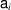ofonto the elements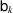ofthat preserves the multiplication relation (in general several elements ofare mapped onto one element of): if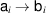and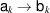, then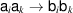holds for all elements ofand(the image of the product is equal to the product of the images). In the special case of a one-to-one mapping, the homomorphism is called an isomorphism.

A matrix group is a group whose elements are non-singular square matrices. The law of combination is matrix multiplication and the group inverse is the inverse matrix. In the following we will be concerned with some basic properties of finite matrix groups relevant to representations.

Letandbe two matrix groups whose matrices are of the same dimension. They are said to be equivalent if there exists a (non-singular) matrix S such thatholds. Equivalence implies isomorphism but the inverse is not true: two matrix groups may be isomorphic without being equivalent. According to the theorem of Schur-Auerbach, every finite matrix group is equivalent to a unitary matrix group (by a unitary matrix group we understand a matrix group consisting entirely of unitary matrices).

A matrix groupis reducible if it is equivalent to a matrix group in which every matrix M is of the formsee e.g. Lomont (1959), p. 47. The groupis completely reducible if it is equivalent to a matrix group in which for all matrices R the submatrices X are O matrices (consisting of zeros only). According to the theorem of Maschke, a finite matrix group is completely reducible if it is reducible. A matrix group is irreducible if it is not reducible.

A (matrix) representationof a groupis a homomorphic mapping ofonto a matrix group. In a representation Γ every element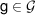is associated with a matrix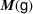. The dimension of the matrices is called the dimension of the representation.

The above-mentioned theorems on finite matrix groups can be applied directly to representations: we can restrict the considerations to unitary representations only. Further, since every finite matrix group is either completely reducible into irreducible constituents or irreducible, it follows that the infinite set of all matrix representations of a group is known in principle once the irreps are known. Naturally, the question of how to construct all nonequivalent irreps of a finite group and how to classify them arises.

Linear representations are especially important for applications. In this chapter only linear representations of space groups will be considered. Realizations and representations are homomorphic images of abstract groups, but not all of them are linear. In particular, the action of space groups on point space is a nonlinear realization of the abstract space groups because isometries and thus symmetry operations of space groupsare nonlinear operations. The same holds for their description by matrix-column pairs (Ww),1 by the general position, or by augmentedmatrices, see IT A, Part 8. Therefore, the isomorphic matrix representation of a space group, mostly used by crystallographers and listed in the space-group tables of IT A as the general position, is not linear.

### References

Lomont, J. S. (1959). Applications of Finite Groups. New York: Academic Press.
Rosen, J. (1981). Resource letter SP-2: Symmetry and group theory in physics. Am. J. Phys. 49, 304–319.